Next: Substitute all non-polynomials. Up: Building additional iterators Previous: Building additional iterators

#### Polynomial iterators.

One of the ways in which we can create new, and hopefully better, iterators is by isolating subexpressions which are easy to invert, instead of isolating x. For example, the equation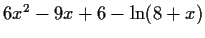generates the iteratorsFor the initial value x0=1.23, all these iterators fail. The first one and the third one grow without bound, the positive second iterator converges too slowly and the negative oscillates between one value and its conjugate. For this equation we could instead isolate the polynomial part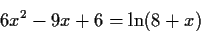solve it treating the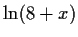as a constant, and obtain two new iterators.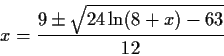Notice that these new iterators have only one occurrence of x on the rhs, compared to two for the previous ones. The positive choice for this iterator is successful and converges very quickly to x=0.7595...+0.2753...i. This is not a general rule, we could certainly find examples which show the contrary, but in general it is expected that such groupings will produce better iterators as they capture more information about the inverse.

So we will use the following rule, for each iterator F(x) which is a sum of a polynomial part and a non polynomial part, i.e.

x = F(x) = G(x) + p(x)

we will produce an iterator which is x-p(x)=G(x) and each iteration consists of evaluatingand then solving the polynomial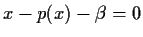. Removing the polynomial part will decrease the number of occurrences of x on the rhs, and hence improve our measure of quality of the iterators. The iterator can be written using Maple's RootOf2 notation asA polynomial iterator, with degree d on its lhs, will normally have d roots. This has a cardinality advantage over handling a polynomial iterator by its individual terms. If the individual terms of the polynomial are isolated one by one, and for each monomial xk, k roots have to be considered, up to d(d+1)/2 iterators could be generated. The drawback is obvious, instead of having a closed form solution for the iterator, we have to solve a polynomial of degree d, and this is typically an iterative process for d>2.

Selecting which root of the polynomial to use is non trivial. When roots are given by a formula, e.g. for d=2, then it is easy to write two iterators, one for each root. But when the roots are found numerically, it is not possible to have a consistent way of using the same root on successive iterations. Actually, the term same root'' does not even make sense for different polynomials, it only makes sense when the roots can be expressed as functions of the coefficients. On the other hand, if the polynomial iterator is converging, we want to select the root which is closest to the iteration value. I.e.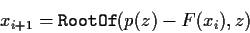and we select the root which is closest to xi. Since this selection is necessary for convergence, we will use it in every step of the algorithm. Furthermore, many iterative methods for solving polynomials can profit significantly from starting their iterators at an approximation of the root; the correct root could come almost for free. For example, for the solution of the equation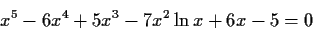we define the iteratorIf we start with x0 = 1, then the rounded roots of the polynomial areThe root which is closest to x0=1 is 0.758845...Hence we set x1=0.758845.... For the second iteration the polynomial to solve, is z5 -6z4 +5z3 +6z -5 =-1.11236...The roots of this polynomial are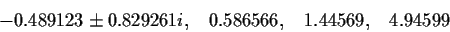and the root closest to x1 is 0.586566... which becomes x2. This process converges quite quickly, x3=0.561733..., x4=0.563302..., x5=0.563168..., etc. This root selection can also be treated as a multiple value function problem, which is discussed in the next section.

Table 2: Iterators which have a non-linear polynomial in their lhs
 66644 random equations producing 59428 iterators method failures converged to a root 44.64% converged to a non-root 1.67% failed 55.36% diverged 21.22% average time 1.593 outside domain 18.06% time per root 3.569 too many iterations 4.79% iterator fails 0.00% iterators per equation .892 no iterators 54.26%

Table 2 shows the simulation results for polynomial lhs iterators. Almost 45% of the iterators yield a root, in time which is of the same order of magnitude as for the simple iterators.Next: Substitute all non-polynomials. Up: Building additional iterators Previous: Building additional iterators
Gaston Gonnet
1998-07-08